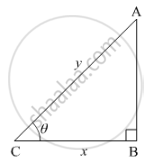Share

# If the sum of lengths of hypotenuse and a side of a right angled triangle is given, show that area of triangle is maximum, when the angle between them is π/3. - CBSE (Commerce) Class 12 - Mathematics

#### Question

If the sum of lengths of hypotenuse and a side of a right angled triangle is given, show that area of triangle is maximum, when the angle between them is π/3.

#### Solution

Let ABC be the right angled triangle with BC = x , AC = y such that x + y = k, where k is any constant. Let θ be the angle between the base and the hypotenuse.Let A be the area of the triangle.

A=1/2xxBC×AC=1/2xsqrt(y^2−x^2)

⇒A^2=x^2/4(y^2−x^2)

⇒A^2=x^2/4[(k−x)^2−x^2]

⇒A^2=(k^2x^2−2kx^3)/4           .....(1)

Differentiating w.r.t. x, we get

2A(dA)/(dx)=(2k^2x−6kx^2)/4       .....(2)

⇒(dA)/(dx)=(k^2x−3kx^2)/(4A)

For maximum or minimum,

(dA)/(dx)=0

⇒(k^2x−3kx^2)/(4A)=0

⇒x=k/3

Differentiating (2) w.r.t. x, we get

2((dA)/(dx))^2+2A(d^2A)/(dx^2)=(2k^2−12kx)/4        .....(3)

Substituting (dA)/(dx)=0 and x=k/3  in (3), we get

(d^2A)/(dx^2)=−k^2/(4A)<0

Thus, A is maximum when x=k/3.

x=k/3⇒y=k−k/3=(2k)/3        [∵x+y=k]

∴ cosθ=x/y

⇒cosθ=(k/3)/((2k)/3)=1/2

⇒θ=π/3

Is there an error in this question or solution?

#### Video TutorialsVIEW ALL 

Solution If the sum of lengths of hypotenuse and a side of a right angled triangle is given, show that area of triangle is maximum, when the angle between them is π/3. Concept: Maxima and Minima.
S